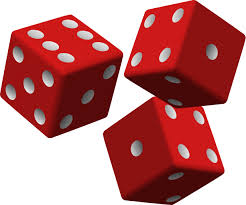# Three dice thrown simultaneously.Three fair cubical dice are thrown. If the probability that the product of the scores on the three dice is $90$ is $\dfrac{a}{b}$, where $a,b$ are positive coprime integers, then find the value of $(b-a)$.

You can try my other Probability problems by clicking here

×